Test: Footprints - 2

# Test: Footprints - 2

Test Description

## 20 Questions MCQ Test Mathematics for Class 2: NCERT | Test: Footprints - 2

Test: Footprints - 2 for Class 2 2023 is part of Mathematics for Class 2: NCERT preparation. The Test: Footprints - 2 questions and answers have been prepared according to the Class 2 exam syllabus.The Test: Footprints - 2 MCQs are made for Class 2 2023 Exam. Find important definitions, questions, notes, meanings, examples, exercises, MCQs and online tests for Test: Footprints - 2 below.
Solutions of Test: Footprints - 2 questions in English are available as part of our Mathematics for Class 2: NCERT for Class 2 & Test: Footprints - 2 solutions in Hindi for Mathematics for Class 2: NCERT course. Download more important topics, notes, lectures and mock test series for Class 2 Exam by signing up for free. Attempt Test: Footprints - 2 | 20 questions in 40 minutes | Mock test for Class 2 preparation | Free important questions MCQ to study Mathematics for Class 2: NCERT for Class 2 Exam | Download free PDF with solutions
 1 Crore+ students have signed up on EduRev. Have you?
Test: Footprints - 2 - Question 1

### Which two shapes are there in the given figure?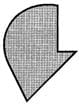Detailed Solution for Test: Footprints - 2 - Question 1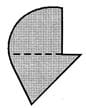The given figure has a quarter circle and a triangle.

Test: Footprints - 2 - Question 2

### Study the given figure and answer the questions that follow.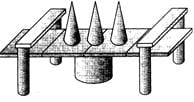Identify the number of cylinders.

Detailed Solution for Test: Footprints - 2 - Question 2

There are 5 cylinders in the given figure.

Test: Footprints - 2 - Question 3

### Study the given figure and answer the questions that follow.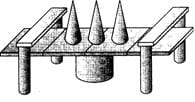How many cones are there?

Detailed Solution for Test: Footprints - 2 - Question 3

There are 3 cones in the figure.

Test: Footprints - 2 - Question 4

Study the given figure and answer the questions that follow.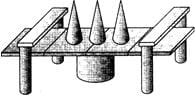How many shapes in all have curved surfaces?

Detailed Solution for Test: Footprints - 2 - Question 4

3 cones and 5 cylinders i.e., 8 shapes have curved surfaces.

Test: Footprints - 2 - Question 5

Find the number of triangles in the figure given.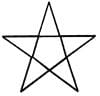Test: Footprints - 2 - Question 6

How many more flat faces does solid A have than Solid B?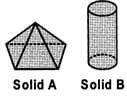Detailed Solution for Test: Footprints - 2 - Question 6

No. of flat faces in Solid A = 5 No. of flat faces in Solid B = 2
∴ Their difference = 5 ? 2 = 3

Test: Footprints - 2 - Question 7

Study the given figure and answer the questions that follow.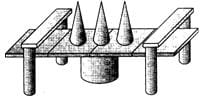How many cuboids are there?

Detailed Solution for Test: Footprints - 2 - Question 7

There are 12 cuboids in the figure.

Test: Footprints - 2 - Question 8

Study the given figure and answer the questions that follow.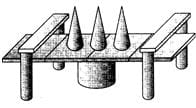How many more cylinders are there than cones?

Detailed Solution for Test: Footprints - 2 - Question 8

There are 3 cones and 5 cylinders in the figure. So, there are 5 - 3 = 2 more cylinders than cones.

Test: Footprints - 2 - Question 9

How many squares are there in the. Given figure?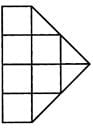Test: Footprints - 2 - Question 10

Find the number of sides in the figure given.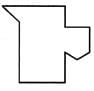Test: Footprints - 2 - Question 11

Which shape is the largest in number in the given figure?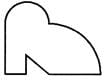Test: Footprints - 2 - Question 12

Identify the shapes in the given figure.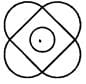Detailed Solution for Test: Footprints - 2 - Question 12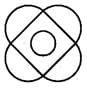The given figure has 4 semicircles, 1 circle and 1 square.

Test: Footprints - 2 - Question 13

How many more squares than circles are there in the figure?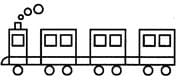Detailed Solution for Test: Footprints - 2 - Question 13

The no. of circles = 10 The no. of squares = 11
∴ There is 1 square more than the number of circles in the given figure.

Test: Footprints - 2 - Question 14

Which shape is 2 more than the number of triangles in the picture?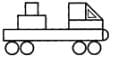Detailed Solution for Test: Footprints - 2 - Question 14

No. of circles = 4 No. of triangles = 2
∴ There are 2 circles more than the number of triangles.

Test: Footprints - 2 - Question 15

How many more rectangular faces than square faces does the solid have?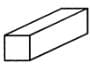Detailed Solution for Test: Footprints - 2 - Question 15

The number of rectangular faces = 4 The number of square faces = 2
∴ The solid has 4 ? 2 = 2 more square faces than rectangular faces.

Test: Footprints - 2 - Question 16

What shapes can be seen in the given figure?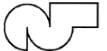Test: Footprints - 2 - Question 17

How many squares are there in the given figure?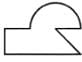Detailed Solution for Test: Footprints - 2 - Question 17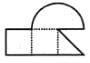Test: Footprints - 2 - Question 18

Find the correct number of each shape in the given figure.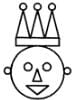Detailed Solution for Test: Footprints - 2 - Question 18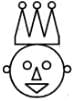The number of circles = 8, the no. of triangles = 5 and the no. of rectangles = 1

Test: Footprints - 2 - Question 19

How many circles are there in the figure given?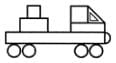Detailed Solution for Test: Footprints - 2 - Question 19

There are 4 circles in the given figure.

Test: Footprints - 2 - Question 20

Identify the correct match of the given parts of shapes.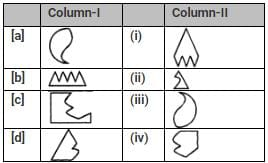Detailed Solution for Test: Footprints - 2 - Question 20

The correct match of the parts of the given shapes is as in option [a].

## Mathematics for Class 2: NCERT

37 videos|62 docs|21 tests
 Use Code STAYHOME200 and get INR 200 additional OFF Use Coupon Code
Information about Test: Footprints - 2 Page
In this test you can find the Exam questions for Test: Footprints - 2 solved & explained in the simplest way possible. Besides giving Questions and answers for Test: Footprints - 2, EduRev gives you an ample number of Online tests for practice

## Mathematics for Class 2: NCERT

37 videos|62 docs|21 tests